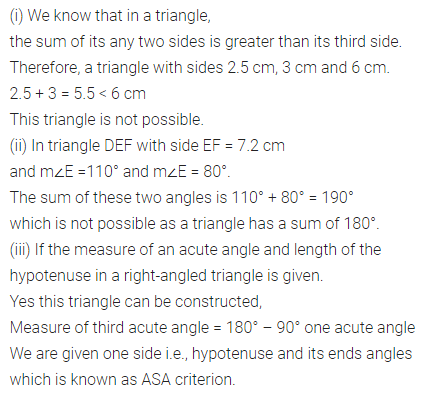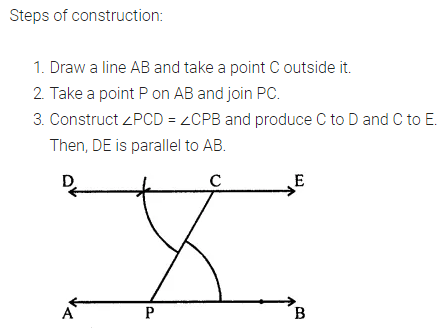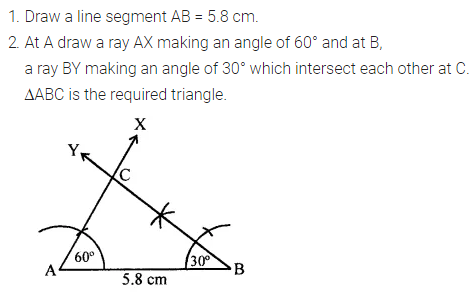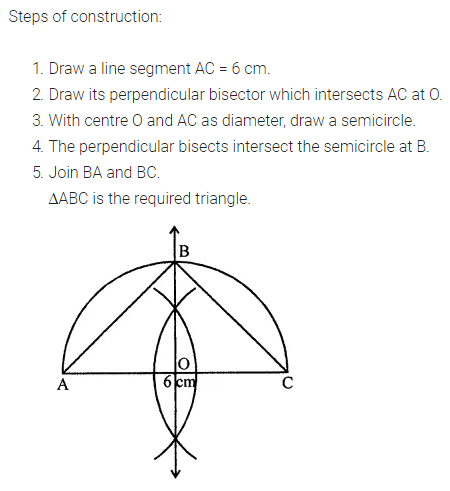ML Aggarwal Class 7 Solutions Chapter 13 Practical Geometry Check Your Progress for ICSE Understanding Mathematics acts as the best resource during your learning and helps you score well in your exams.

## ML Aggarwal Class 7 Solutions for ICSE Maths Chapter 13 Practical Geometry Check Your Progress

Question 1.
State whether the following statements are true or false. Justify your answer.
(i) A triangle with lengths of sides 2.5 cm, 3 cm, and 6 cm can be constructed.
(ii) A triangle DEF with EF = 7.2 cm, m∠E = 110° and m∠F = 80° can be constructed.
(iii) If the measure of an acute angle and the length of the hypotenuse of a right-angled triangle are given, then the triangle can be constructed.
Solution:Question 2.
Draw a line AB and take a point C outside it. Through C, draw a line parallel to AB by using the concept of equal corresponding angles.
Solution:Question 3.
Draw a triangle PQR with PQ = 4 cm, QR = 3.5 cm and PR = 4 cm. What type of triangle is this?
Solution:Question 4.
Construct a triangle ABC with BC = 7.5 cm, AC = 5 cm and m∠C = 60° by using ruler and compasses only.
Solution:Question 5.
Construct a triangle ABC, given m∠A = 60°, m∠B = 30° and AB = 5.8 cm by using ruler and compasses only.
Solution:
Steps of Construction:Question 6.
Construct an isosceles right angled triangle ABC, with m∠ABC = 90° and AC = 6 cm.
Solution: